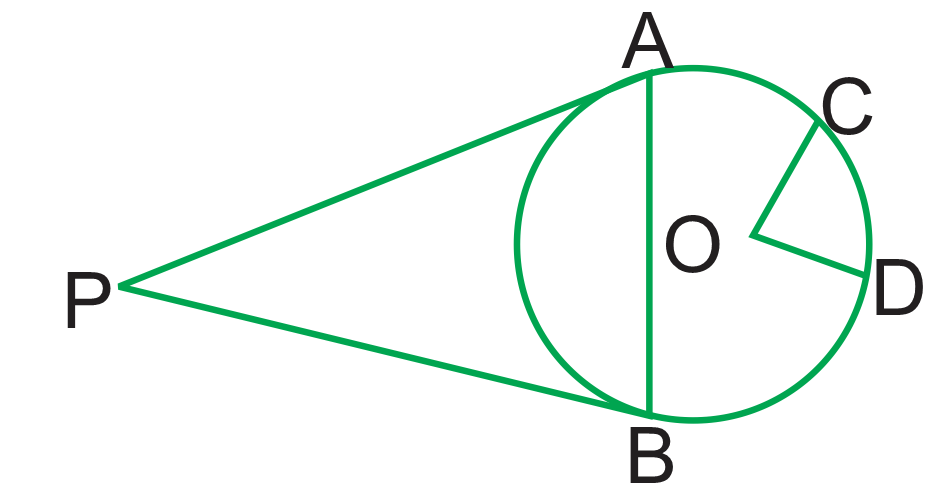• Save

# Geometry Questions for Competitive Exams

Gajanand2 years ago 18.2K ViewsQ :

Two parallel chords are drawn in a circle of diameter 30 cm. The length of one chord is 24 cm and the distance between the two chords is 21 cm. The length of the other chord is 30 cm

(A) 10 cm

(B) 18 cm

(C) 12 cm

(D) 16 cm

Correct Answer : B

Q :

In the given figure, from the point P two tangents PA and PB are drawn to a circle with center O and radius 5 cm. From the point O, OC and OD are drawn parallel to PA and PB respectively. If the length of the chord AB is 5 cm. then what is the value (in degrees) of ∠COD?(A) 90

(B) 120

(C) 150

(D) 135

Correct Answer : B

Q :

The area of a triangle with vertices A (0, 8), O (0, 0), and B (5, 0) is :

(A) 8 sq. units

(B) 13 sq. units

(C) 20 sq. units

(D) 40 sq. units

Correct Answer : C

Q :

The equidistant point from the vertices of a triangle is called its:

(A) Centroid

(B) Incentre

(C) Circumcenter

(D) Orthocenter

Correct Answer : C

Q :

AD is the median of a triangle ABC and O is the centroid such that AO = 10 cm. The length of OD (in cm) is

(A) 14

(B) 5

(C) 6

(D) 8

Correct Answer : B

Q :

In triangle PQR, points A, B and C are taken on PQ, PR and QR respectively such that QC = AC and CR = CB. If ∠QPR = 40°, then ∠ACB is equal to:

(A) $$140^°$$

(B) $$40^°$$

(C) $$70^°$$

(D) $$100^°$$

Correct Answer : D

Q :

O is the center and are ABC subtends an angle of 130at O. AB is extended to P, then ∠PBC is

(A) $$75^°$$

(B) $$70^°$$

(C) $$65^°$$

(D) $$80^°$$

Correct Answer : C

### Gajanand

B-Tech Electronic and Communication Engineering select the SSC CGL Tier-1 and Tier-2 in 2016 and Rajasthan state Govt. Exams Expert in Mathematics , Reasoning Gk and English.

## Report Error: Geometry Questions for Competitive Exams